﻿ Kinetics of Recovery of Alumina from Coal Fly Ash through Fusion with Sodium Hydroxide

### Kinetics of Recovery of Alumina from Coal Fly Ash through Fusion with Sodium Hydroxide

M A Rahaman, M A Gafur, A S W KurnyOPEN ACCESSPEER-REVIEWED

## Kinetics of Recovery of Alumina from Coal Fly Ash through Fusion with Sodium Hydroxide

M A Rahaman1, M A Gafur1, A S W Kurny1,1Department of Materials and Metallurgical Engineering, Bangladesh University of Engineering and Technology, Dhaka, Bangladesh

### Abstract

Coal fly ash containing 35.9 % Al2O3 and 53.0%SiO2 was fused with NaOH for a period of up to 60 minutes in the temperaure range of 300°C-800°C in a stainless steel crucible. The fused mass was dissolved in water. Amount of alumina in the solution was determined by by EDTA compleximetric (masking and demasking) chemical method. The optimum condition for alumina extraction was determined as NaOH to CFA weight ratio = 3.5, temperature = 700°C and time = 30 minutes. Avrami-Erofeev equation i.e. –ln(1-α) = (k3t) m (when m=1) seemed to be the most appropriate one to fit the kinetic data of fusion of coal fly ash with NaOH. The activation energy was found to be 13.94 kJ/mol.

### At a glance: Figures

123
Prev Next

• Rahaman, M A, M A Gafur, and A S W Kurny. "Kinetics of Recovery of Alumina from Coal Fly Ash through Fusion with Sodium Hydroxide." American Journal of Materials Engineering and Technology 1.3 (2013): 54-58.
• Rahaman, M. A. , Gafur, M. A. , & Kurny, A. S. W. (2013). Kinetics of Recovery of Alumina from Coal Fly Ash through Fusion with Sodium Hydroxide. American Journal of Materials Engineering and Technology, 1(3), 54-58.
• Rahaman, M A, M A Gafur, and A S W Kurny. "Kinetics of Recovery of Alumina from Coal Fly Ash through Fusion with Sodium Hydroxide." American Journal of Materials Engineering and Technology 1, no. 3 (2013): 54-58.

 Import into BibTeX Import into EndNote Import into RefMan Import into RefWorks

### 1. Introduction

Commercial alumina is produced by the Bayer process using bauxite as raw material. Alternative methods for alumina extraction from different source materials such as discarded alumina bricks , spent catalyst , clays and kaolins [3, 4], coal fly ash , etc. have also been developed. Low aluminum contents of coal fly ash make the Bayer process unsuitable for extraction of alumina from it.

Technologies of alumina extraction from coal fly ash can be classified into acidic, alkali, and acid-alkali methods [6-11]. The acidic method uses sulfuric acid , hydrochloric acid or hydrofluoric acid . These methods require acid-resistant and air-tight processing equipment. In addition, alumina recovery and waste disposal are also very complex.

Alkali methods use lime and/or soda in sintering with coal fly ash [8, 9, 10, 11]. BAI Guang-hui  reported an alkali process in which desilicated fly ash was mixed with lime and soda and then sintered. The sintered product was dissolved in NaOH solution at different temperature and stirring speed. Lu et al  reported an alkali process in which coal fly ash containing 26.38% alumina and 52.10% silica was mixed with limestone, sintered, and then dissolved in sodium carbonate solution.

In an acidic-alkali method coal fly ash was sintered with soda, and then aluminum as aluminum chloride and silica as silica gel were recovered, with diluted hydrochloric acid . Similar to other two methods, the acid-alkali method also faces with some problems such as massive consumptions of strong acid and caustic base and difficulty in separation of alumina from iron and titanium impurities .

### 2. Experimental

2.1. Materials Composition and Phase Identification

The coal fly ash (CFA) for this study was collected from Barapukuria Thermal Power Plant located in Northern Bangladesh. The chemical composition of the fly ash sample as determined by energy dispersive x-ray fluorescence (ED-XRF) is given in Table 1.

X-ray diffraction pattern of fly ash sample used in this study (Figure 1) showed that the major phases present in the sample were quartz and sillimanite.

#### Table 1. Chemical composition of coal fly ash raw material used in this study (mass fraction, %)Download asPowerPoint Slide

Veiw figureView current table in a new window

Figure 1. X-ray diffraction pattern of fly ash (S = sillimanite, Q = quartz)
2.2. Fusion Procedure and % Alumina Estimation

2g of fly ash sample was mixed with a specified amount of sodium hydroxide (NaOH). The mixed sample was placed in a stainless steel crucible, covered with cap and placed in a muffle furnace. The fused sample was cooled to room temperature and dissolved in distilled water. The solution was filtered and the amount of alumina in the solution was determine by EDTA compleximetric (masking and demasking) chemical method.The effects of NaOH to CFA weight ratio in the range 0.75 – 4.0, temperature in the range 300 – 800°C and time of fusion upto 60 minutes on the extent of alumina recovery were studied. The activation energy of fusion was determined and a kinetic model was also fitted for the fusion process.

### 3. Results and Discussion

3.1. Optimization of NaOH to CFA Weight Ratio

Effects of sodium hydroxide (NaOH) to fly ash ratio (weight basis) in the range 0.75 - 4 were studied. The extent of extraction of alumina was found to increase with an increase in concentration of sodium hydroxide in the fusion mixture up to a sodium hydroxide (NaOH) to fly ash ratio of 3.5 (Figure 2). This sodium hydroxide to fly ash ratio was taken to be the optimum and was kept constant in the subsequent experiments.

Figure 2. Plot of %Al extracted versus NaOH to CFA wt. ratio (fusion time = 2 h, Temperature = 800°C)

3.2. Optimization of Temperature

Effect of temperature in the range 300- 800°C on the extent of extraction at a constant NaOH to CFA weight ratio 3.5 and time 2h is shown in Figure 3. It can be seen that the extent of extraction increased with an increase in reaction temperature up to 700°C. Fly ash-sodium hydroxide mixture fused above 800°C did not dissolve in water and the extent of fusion at temperatures above 800°C could not be determined.

Figure 4. Plot of %Al extracted versus time (at NaOH:CFA = 3.5, Temperature = 700°C)

3.3. Optimization of Time

To optimise time of fusion NaOH-CFA mixture was fused by using the optimised parameters of fusion (temperature= 700°C, NaOH/CFA ratio = 3.5). It was found that maximum yield reached within 30 minutes (Figure 4).

### 4. Identification of Reaction Model

In systems involving solid reactants, the chemical reaction takes place at the interface of the reactants to produce a final compound . Diffusion must then occur through the product for the reaction to continue and the chemical reaction will proceed at the interface of one or both of the reactants. The mechanism of these steps will depend upon the experimental conditions and upon the characteristics of the solid reactants; therefore, the overall rate of the process may be controlled by chemical kinetics or transport processes.

The Kinetic Models are considered for the recent study are given bellow 

1. Parabolic equation: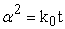(1)

2. Ginstling and Brounshtein: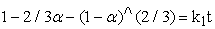(2)

3. Jander equation: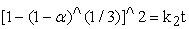(3)

4. Avrami-Erofeev equation: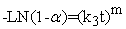(4)

Where,

α= fraction of reacted particles

t = time for fraction of reacted particles

ki = specific rate constant

m= parameter which depends on geometry

Intergal method (reduced time plot) was used to determine the model of the reaction. From Figure 5 times for different fractions of reacted particle of alumina were calculated at 300°C, 500°C and 600°C. Then degree of reaction versus time/time at α=0.15 for comonly used solid state reaction model (Figure 6) was plotted. Then a diagram with the same co-ordination on the same diagram from the actual experimental data also plotted. From this plot it was evaluated that, the actual experimental data only best match with the Avrami-Erofeev equation (-LN (1-α) = (k3t) m) for m=1. So the Avrami-Erofeev equation when m=1 was taken as the reaction model for extracting alumina from coal fly ash (CFA) by NaOH fusion method. Kinetic plots for Avrami-Erofeev equation (-LN (1-α) = (k3t)m ) model equations for m=1 was shown on Figure 7. This plot yielded a very high correlation at all temperatures under this investigation. Moreover, the plotted lines passed through the zero point. When m equal to 1 Avrami-Erofeev equation become first order reaction. Therefore, the data could be correlated to a First order reaction mechanism also.

### 5. Evaluation of Activation Energy

Both the conventional integral approach and differential approach were used to evaluate the activation energy of the fusion process.

In the integral approach the relationship between the rate constant k and temperature is given by the Arrhenius equation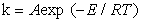where A is the frequency factor and E is the apparent activation energy. Apparent activation energy determined from the Arrhenius type plots (Figure 8), where - lnK. Versus 1/T is plotted for F1 model. Slope of the plot gave E/R and activation energy found to be 13.94 kJ/mol.

In the differential approach activation energy was determined in three different extraction levels (α) i.e. α = 0.1, 0.15 and 0.20 of alumina by differential approach. Time of attaining different extraction level at different temperatures are cross plotted in Figure 9. From this figure the value k (= E/T) which is equal to the slope of ln(tα) versus 1/T plot..The Activation energy evaluated at α = 0.1, 0.15 and 0.2 ware 14.47, 13.58 and 13.40 kJ/mol respectively.

Figure 9. Plots of ln(tα) versus 1/T to determine activation energy (differential approach)

### 6. Conclusions

The maximum extraction of alumina was obtained at NaOH to coal fly ash ratio of 3.5 and 700o C within 30 minute. The rate of alumina extraction followed Avrami-Erofeev equation: -LN (1-α) = (k3t)m when, m= 1(first order reaction mechanism) with apparent activation energy of about13.94 kJ/mol.

### Acknowledgements

The authors are grateful to the authorities of Pilot Plant and Process Development Centre, Bangladesh Council for Industrial and Scientific Research for permission to use their facilities and to the authorities of Bangladesh University of Engineering and Technology for providing the financial support for this study.

### References

  N A Siddique and A S W Kurny, “Kinetics of leaching of alumina from discarded high alumina refractory bricks”, International Journal of Engineering & Technology IJET-IJENS Vol: 10 No: 01, pp 23-26.In article  Abdel-Aal E.A., Rashad M.M., “Kinetic study on the leaching of spent nickel oxide catalyst with sulfuric acid”, Hydrometallurgy, Vol. 74, pp 189-194, 2004.In article CrossRef  U.K.Sultana, A.S.W.Kurny., “Kinetics of dissolution of iron oxide in clay in hydrochloric acid solutions”, Physical CHEMISTTRY, PCAIJ, 7(2), 63-67, 2012.In article  Bazin C., El-Quassirri K., Uuellet V., “Sequential leaching for the recovery of alumina from a Canadian clay”, Hydrometallurgy, Vol. 88, pp 196-201, 2007.In article CrossRef  Felker K., Seeley F., Egan Z., Kelmers D., “Aluminum from fly ash”, ChemTech, Vol. 12, pp 123, 1982.In article  SEIDEL A, SLUSZNY A, SHELEF G, ZIMMELS Y. Self inhibition of aluminum leaching from coal fly ash by sulfuric acid[J]. Chemical Engineering Journal, 1999, 72: 195-207.In article CrossRef  KUMAMOTO J. Recovery of metal oxides from fly ash. Kobelco Technology Review, 1990(7): 53-57.In article  PADILLA R, SOHN H Y. Sintering Kinetics and Alumina Yield in lime-soda sinter process for alumina from coal wastes [J]. Metallurgical Transactions B, June- 1985, Volume 16B: 385.In article  MATJIE R H, BUNT J R, and HEERDEN J H P. Minerals Engineering, 2005, 18: 299-310.In article  BAI Guang-hui, et al/Trans. “Alkali desilicated coal fly ash as substitute of bauxite in lime-soda sintering process for aluminum production”, Nonferrous Met. Soc. China 20(2010) s169-s175.In article CrossRef  LU S, FANG R L, ZHAO H. Study of recovery of highly pure super-fine powdered aluminum oxide from fly ash by way of lime sintered self powdering [J]. Scientific Study, 2003(1): 15-17.In article  VIKTOR L R, SOLOMON A S, RONALD S D. Technology for chemical-metallurgical coal ash utilization [J]. Energy & Fuels, 1997, 11: 761-773.In article CrossRef